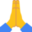Android ExampleAnalog Clock with Sound

Hi!

Since I've been messing around with trigonometry this week, I decided to give you guys an analog clock example. Enjoy!

B4X:
#Region  Project Attributes
#ApplicationLabel: Analog Clock
#VersionCode: 1
#VersionName: 1
'SupportedOrientations possible values: unspecified, landscape or portrait.
#SupportedOrientations: unspecified
#CanInstallToExternalStorage: False
#End Region

#Region  Activity Attributes
#FullScreen: True
#IncludeTitle: False
#End Region

Sub Process_Globals
Dim TicTac As Timer
End Sub

Sub Globals

Dim Wall As Canvas

Dim maxresX = GetDeviceLayoutValues.Width  As Float
Dim maxresY = GetDeviceLayoutValues.Height As Float

Dim Clock_X, Clock_Y As Float

Dim Hour_Handle_Size, Mins_Handle_Size, Secs_Handle_Size As Float

Dim Sound   As SoundPool
Dim ticksnd As Int

End Sub

Sub Activity_Create(FirstTime As Boolean)

'Clock Position on the wall
Clock_X = maxresX / 2
Clock_Y = maxresY / 2

'Resize the clock accordingly to the screen orientation
If maxresX > maxresY Then
Else
End If

'Set the handle size accordingly to the clock size

'Initialize the Sound
Sound.Initialize(2)

'Initialize the Wall
Wall.Initialize(Activity)

'Initialize the Clock
TicTac.Initialize("TicTac", 1000)
TicTac.Enabled = True

End Sub

Sub TicTac_Tick
'Redraw everything
Activity.Invalidate

'Get the current time
Dim Hour = DateTime.GetHour(DateTime.Now)   As Int
Dim Mins = DateTime.GetMinute(DateTime.Now) As Int
Dim Secs = DateTime.GetSecond(DateTime.Now) As Int

'Brace yourselves. Math is coming!
Dim Hour_X, Hour_Y, Mins_X, Mins_Y, Secs_X, Secs_Y As Float
Dim Hour_Angle, Mins_Angle, Secs_Angle             As Float

'270 degrees is the position for midnight
Hour_Angle = 270 + (Hour * 360 / 12)
Mins_Angle = 270 + (Mins * 360 / 60)
Secs_Angle = 270 + (Secs * 360 / 60)

Hour_X = (CosD(Hour_Angle) * Hour_Handle_Size) + Clock_X
Hour_Y = (SinD(Hour_Angle) * Hour_Handle_Size) + Clock_Y

Mins_X = (CosD(Mins_Angle) * Mins_Handle_Size) + Clock_X
Mins_Y = (SinD(Mins_Angle) * Mins_Handle_Size) + Clock_Y

Secs_X = (CosD(Secs_Angle) * Secs_Handle_Size) + Clock_X
Secs_Y = (SinD(Secs_Angle) * Secs_Handle_Size) + Clock_Y

'Draw the clock
Draw_Clock

'Draw the handles on the clock
Wall.DrawLine(Clock_X, Clock_Y, Hour_X, Hour_Y, Colors.Blue,  Clock_Radius * 0.03)
Wall.DrawLine(Clock_X, Clock_Y, Mins_X, Mins_Y, Colors.Green, Clock_Radius * 0.03)
Wall.DrawLine(Clock_X, Clock_Y, Secs_X, Secs_Y, Colors.Red,   Clock_Radius * 0.01)
Sound.Play(ticksnd, 1, 1, 1 , 0, 0)
End Sub

Sub Draw_Clock

'Draw the clock itself
Wall.DrawCircle(Clock_X + 1%x, Clock_Y + 1%y, Clock_Radius, Colors.Gray, True, 0)
Wall.DrawCircle(Clock_X, Clock_Y, Clock_Radius, Colors.White, True, 0)
Wall.DrawCircle(Clock_X, Clock_Y, Clock_Radius * 0.05, Colors.Black, True, 0)

'Draw the 12 dots representing the hours
Dim midnight = 270 As Int
For angle = midnight To (midnight + 360) Step (360 / 12)
Dim x = (CosD(angle) * Clock_Radius * 0.80) + Clock_X As Float
Dim y = (SinD(angle) * Clock_Radius * 0.80) + Clock_Y As Float
Wall.DrawCircle(x, y, Clock_Radius * 0.05, Colors.Black, True, 0)
Next
End Sub

Sub Activity_Resume

End Sub

Sub Activity_Pause (UserClosed As Boolean)

End Sub

If you need anything to be explained in detail, just let me know. I'll be glad to help.PS: If you'd rather copy-paste the code, you'll need the Sound lib and an mp3 file named "tick.mp3" in your assets folder.

Attachments

• analog.zip
9.9 KB · Views: 798
••Alexander Stolte, Marvel, Procesor and 9 others

Peter Simpson

Expert
Nicely though out bit of code @wonderCheers...

wonder

Expert
Thanks!!I hope someone finds this useful, especially for smart watches.

wonder

Expert
Just for kicks, here's the QBasic version!B4X:
CLS
SCREEN 12

DO WHILE INKEY\$ = ""

IF TIME\$ <> t\$ THEN CLS

pi = 3.1415

ox = 320
oy = 240

t\$ = TIME\$
h = VAL(t\$)
m = VAL(MID\$(t\$, 4, 2))
s = VAL(MID\$(t\$, 7, 2))

ha = (270 + (h * 360 / 12)) * pi / 180
ma = (270 + (m * 360 / 60)) * pi / 180
sa = (270 + (s * 360 / 60)) * pi / 180

hx = (COS(ha) * 30) + ox
hy = (SIN(ha) * 30) + oy

mx = (COS(ma) * 50) + ox
my = (SIN(ma) * 50) + oy

sx = (COS(sa) * 50) + ox
sy = (SIN(sa) * 50) + oy

CIRCLE (ox, oy), 70, 15
LINE (ox, oy)-(hx, hy), 15
LINE (ox, oy)-(mx, my), 15
LINE (ox, oy)-(sx, sy), 12

LOOP

Last edited:

Peter Simpson

Expert
Loaded it into QB64, works a treat...

•wonder

afu

New Member
wow.I like it.and will study from it. thankyou!

•wonder

Replies
0
Views
167
Replies
2
Views
300
Share My Creation B4A Clock 24 Widget
Replies
4
Views
2K
Android Example Another Analog Clock
Replies
8
Views
5K
Replies
4
Views
4K